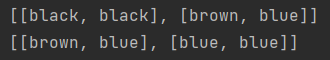×

Search anything:

# Three - Dimensional 3D ArrayList in Java

#### JavaGet this book -> Problems on Array: For Interviews and Competitive ProgrammingIn this article, we have explored the concept of 3D ArrayList in Java and demonstrated how to perform different operations such as insert, delete, search and much more with Java code snippets.

## Why do we need arrays?

Suppose you want to store five integer numbers. What will you do? You will create five different variables and store interger numbers in them. Now let's say you want to store 10000 integer numbers. Is it feasible to create 10000 variables for this? Not at all! To handle these situations, in almost all programming language we have a concept called `array`.

Array is a data structure used to store a collection of data.

Syntax of an array:
`datatype[] variableName = new datatype[size];`
For example, we want to store roll numbers:
`int[] rollNo = new int`
Here int represent the type of the data stored in array. All the type of data in the array should be same.

## Why we need an ArrayList?

Arrays are of fixed size. You have to give a size. If you don't specify the size of array, then compiler will give error. What if you don't know how much size you need? In that case you have to use `ArrayList`.

ArrayList is a resizable array and a part of collection framework and can be found in java.util.package. It provides us with dynamic arrays in Java. It is slower than standard arrays. Elements can be added or removed from an ArrayList whenever you want. ArrayList is a class.

Syntax of an ArrayList
`ArrayList<ArrayList<Object>> object_name = new ArrayList<ArrayList<Object>>();`

Two-Dimensional ArrayList
Suppose you want to represent a graph with 4 vertices, numbered 0 to 3. You can create a 2D ArrayList by creating an ArrayList of ArrayLists.
For example:-
`ArrayList<ArrayList<Integer>> graph = new ArrayList<>(vertexCount);`

You can initialize each element of ArrayList with another ArrayList:

``````for (int i = 0; i < vertexCount; i++) {
}
``````

Example:

``````// Example of Two-Dimensional ArrayList

import java.util.*;

class TwoDimensionalArrayList {

static List create2DArrayList()
{

// Creating a 2D ArrayList of Integer type
ArrayList<ArrayList<Integer> > x= new ArrayList<ArrayList<Integer> >();

// This method will return 2D array
return x;
}

public static void main(String args[])
{
System.out.println("2D ArrayList :");

System.out.println(create2DArrayList());
}
}
``````

## Three-Dimensional ArrayList

In the previous section, we created a two - dimensional ArrayList. Following the same logic, we can create a three-dimensional ArrayList.

Suppose we want to represent a 3-d space. Each point in 3-D space will be represented by three coordinates, x, y and z. Suppose, each of these points will have a color, blue, black or brown. Now, each point (x, y, z) and its color can be represented by a three-dimensional ArrayList.

To keep it simple, let's create (2 x 2 x 2) 3-D space. It will contain total eight points: (0, 0, 0), (0, 0, 1), (0, 1, 0), (1, 0, 0), (0, 1, 1), (1, 0, 1), (1, 1, 0), (1, 1, 1).

First initialize the variables and 3-D ArrayList:

``````int xLength = 2;
int yLength = 2;
int zLength = 2;

ArrayList<ArrayList<ArrayList<String>>> space = new ArrayList<>(xLength);
``````

Now, initialize each element of ArrayList with `ArrayList<ArrayList<String>>`;

``````for (int i = 0; i < xLength; i++){
for (int j = 0; j < yLength; j++){
}
}
``````

Now add colours to the points in the space created. Let's add black for points (0, 0, 0) and (0, 0, 1):

``````    space.get(0).get(0).add(0, "black");
``````

Add brown for points (0, 1, 0) and (1, 0, 0):

``````    space.get(0).get(1).add(0, "brown");
``````

Similarly, by this way we can continue to add data for other points.
`Note:` A point with coordinates (i, j, k), has its colour information stored in the following ArrayList element:

``````   space.get(i).get(j).get(k)
``````

The combined code for the above example is given below:

``````import java.util.ArrayList;

public class Main {
public static void main(String[] args) {

int xLength = 2;
int yLength = 2;
int zLength = 2;

ArrayList<ArrayList<ArrayList<String>>> space = new ArrayList<>(xLength);

for (int i = 0; i < xLength; i++) {
for (int j = 0; j < yLength; j++) {
}
}

for (int i = 0; i < space.size(); i++) {
System.out.println(space.get(i));
}
}
}
``````

In this example, the space variable is an ArrayList. And each element of this ArrayList is a 2-D ArrayList. The index of elements in space ArrayList represents the x-coordinate, while each 2-D ArrayList (at that index), represents the y and z coordinates.

Output:## Methods of ArrayList Class

To add an element to the arraylist you can use `add()` method of the `ArrayList` Class.
In the above example, we have added elements to the ArrayList using add() method:-

`````` space.get(0).get(0).add(0, "black");
``````

2) Access elements of ArrayList
To access the elements of the arraylist you can use `get()` method of the `ArrayList` Class.
In the above example, we have accessed the ArrayList element using get() method.

``````space.get(i);
``````

3) Remove elements of ArrayList
To remove the elements of the arraylist you can use `remove()` method of the `ArrayList` Class.
In the above example, we can remove any element using remove() method.
For example:-

``````space.get(0).get(0).remove(0, "black");
``````

4) Change elements of ArrayList
To change the elements of the arraylist you can use `set()` method of the `ArrayList` Class.
In the above example, we can change any element using set() method.
For example:-

``````space.get(0).get(0).set(0, "brown");
``````

This will change colour from black to brown.

### Some other methods of ArrayList Class

size():- Returns the length of the ArrayList

sort():- Sorts the elements of the ArrayList.

contains():- Checks if the specified elements is in the arraylist and returns a boolean result.

isEmpty():- Checks if the ArrayList is empty

indexOf():- Checks the specified element in the ArrayList and returns the index of the element.

With this article at OpenGenus, you must have the complete idea of 3D ArrayList in Java. Enjoy.